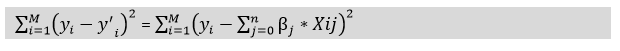# Regularization in Machine Learning

## What is Regularization?

Regularization is one of the most important concepts of machine learning. It is a technique to prevent the model from overfitting by adding extra information to it.

Sometimes the machine learning model performs well with the training data but does not perform well with the test data. It means the model is not able to predict the output when deals with unseen data by introducing noise in the output, and hence the model is called overfitted. This problem can be deal with the help of a regularization technique.

This technique can be used in such a way that it will allow to maintain all variables or features in the model by reducing the magnitude of the variables. Hence, it maintains accuracy as well as a generalization of the model.

It mainly regularizes or reduces the coefficient of features toward zero. In simple words, “In regularization technique, we reduce the magnitude of the features by keeping the same number of features.”

## How does Regularization Work?

Regularization works by adding a penalty or complexity term to the complex model. Let’s consider the simple linear regression equation:

`y= β0+β1x1+β2x2+β3x3+⋯+βnxn +b`

In the above equation, Y represents the value to be predicted

X1, X2, …Xn are the features for Y.

β0,β1,…..βn are the weights or magnitude attached to the features, respectively. Here represents the bias of the model, and b represents the intercept.

Linear regression models try to optimize the β0 and b to minimize the cost function. The equation for the cost function for the linear model is given below:Now, we will add a loss function and optimize parameter to make the model that can predict the accurate value of Y. The loss function for the linear regression is called as RSS or Residual sum of squares.

## Techniques of Regularization

There are mainly two types of regularization techniques, which are given below:

• Ridge Regression
• Lasso Regression

## Ridge Regression

• Ridge regression is one of the types of linear regression in which a small amount of bias is introduced so that we can get better long-term predictions.
• Ridge regression is a regularization technique, which is used to reduce the complexity of the model. It is also called as L2 regularization.
• In this technique, the cost function is altered by adding the penalty term to it. The amount of bias added to the model is called Ridge Regression penalty. We can calculate it by multiplying with the lambda to the squared weight of each individual feature.
• The equation for the cost function in ridge regression will be:
•• In the above equation, the penalty term regularizes the coefficients of the model, and hence ridge regression reduces the amplitudes of the coefficients that decreases the complexity of the model.
• As we can see from the above equation, if the values of λ tend to zero, the equation becomes the cost function of the linear regression model. Hence, for the minimum value of λ, the model will resemble the linear regression model.
• A general linear or polynomial regression will fail if there is high collinearity between the independent variables, so to solve such problems, Ridge regression can be used.
• It helps to solve the problems if we have more parameters than samples.

## Lasso Regression:

• Lasso regression is another regularization technique to reduce the complexity of the model. It stands for Least Absolute and Selection Operator.
• It is similar to the Ridge Regression except that the penalty term contains only the absolute weights instead of a square of weights.
• Since it takes absolute values, hence, it can shrink the slope to 0, whereas Ridge Regression can only shrink it near to 0.
• It is also called as L1 regularization. The equation for the cost function of Lasso regression will be:
•• Some of the features in this technique are completely neglected for model evaluation.
• Hence, the Lasso regression can help us to reduce the overfitting in the model as well as the feature selection.

## Key Difference between Ridge Regression and Lasso Regression

• Ridge regression is mostly used to reduce the overfitting in the model, and it includes all the features present in the model. It reduces the complexity of the model by shrinking the coefficients.
• Lasso regression helps to reduce the overfitting in the model as well as feature selection.

Thank You
Mahendran M
Helical IT SolutionsBest Open Source Business Intelligence Software Helical Insight is Here